# Quiz 24: The Monetary System

Business

Aggregate Expenditure Aggregate expenditure is the sum of total spending at each level of income for a given price level during a particular time period. In general, aggregate expenditure is defined as the sum of expenditures on consumption, investment, government expenses, and net exports. Components of aggregate expenditure Aggregate expenditure comprises the following components: • Household final consumption expenditure.• Gross private domestic investment. • Government final consumption expenditure.• Net exports. Household final consumption expenditure Household final consumption expenditure is the total expenditure incurred by all the households of an economy to purchase final goods and services for private uses. Gross private domestic investment Gross private domestic investment is the sum of gross domestic fixed capital formation and change in the net inventories, during a given period.Government final consumption expenditure Government final consumption expenditure is the total expenditure incurred by the government of an economy to purchase final goods and services to meet internal and external security, social security etc.Net exports Net export is defined as the difference between a country's exports minus imports at a given period.Components of aggregate expenditure and change in level of income More general aggregate income-expenditure model assumes that the investment, government expenses, and net exports are independent of the change in level of income.Therefore, change in the level of income would change the aggregate expenditure only by altering the level of consumption expenditure incurred by the households. So, the slope of the aggregate expenditure line will be subject to change in the consumption expenditure of households. Change in the consumption expenditure due to change in income can be tracked by the marginal propensity to consume, which is the slope of the consumption expenditure.Derivation of the slope of Aggregate Expenditure Assume that the investment, government expenses, and net exports are independent of change in the level of income. Then, the aggregate income-expenditure model can be written as follows.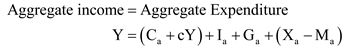Where,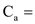autonomous consumption expenditure.c = marginal propensity to consume.Y = disposable income.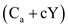= consumption expenditure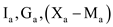= autonomous investment, government spending, and net exports, respectively. To solve for the level of income, the aggregate income-expenditure model can be obtained as follows: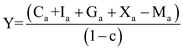…… (1)Equation (1) is differentiated with respect to the level of income to obtain the slope of the aggregate expenditure function.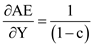Thus, the slope of the aggregate expenditure curve is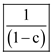.

Aggregate Income-Expenditure Model Assuming there is no depreciation of capital stock or no fall in net inventories and no savings of firms, aggregate income-expenditure model states that each dollar of expenditure on final goods and services results in a dollar of income.In simple words, income-expenditure model states that when there is no depreciation of capital stock and no business saving, then an economy's aggregate expenditure results in aggregate income, which is equal to the real gross domestic product (Real GDP). Determination of Real GDP demanded Real GDP is determined at the intersection point between the aggregate expenditure line and the 45-degree line. The 45-degree line reflects the locus of points where aggregate income is equal to the real GDP. In simple words, income-expenditure model states that at the given price level, real GDP demanded is determined where the aggregate expenditure and real GDP are equal. Real GDP can be stated as the aggregate output or aggregate income. Real GDP is measured on horizontal axis and aggregate expenditure is measured on vertical axis. Below the intersection point, desired spending is more than the real GDP and above the intersection point, real GDP is more than the desired spending.

Real GDP demanded and aggregate Demand-Supply Identity Equilibrium of an economy occurs where aggregate demand is equal to the aggregate supply. Thus, at equilibrium, aggregate demand-supply identity holds. Aggregate demand-supply identity is also known as aggregate expenditure-income identity. At the point of equilibrium, real GDP demanded equals real GDP or aggregate output produced.Aggregate Demand-Supply Identity Aggregate demand-supply identity can be written as follows: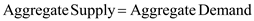or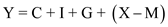or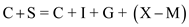Where, Y = Level of income or money supply in an economy. C = Consumption expenditure of households. S = Savings by households. I = Investment. G = Government Spending.(X-M) = Net exports. Assumptions: Aggregate Demand-Supply Identity At the equilibrium, real GDP demanded equals real GDP, keeping price level constant. But inventories of firms vary according to the operational situations of firms. If firms are operating at the level of output which (real GDP) is less than the real GDP demanded, that is, when firms are under-producing, then those firms will expand their stock of inventories to meet the actual real GDP demanded.Similarly, when firms are over-producing than what they require to meet the real GDP demanded, then the firms will reduce their inventory holdings.